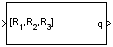# Rotation Angles to Quaternions

Calculate quaternion from rotation angles

•Libraries:
Aerospace Blockset / Utilities / Axes Transformations

## Description

The Rotation Angles to Quaternions block converts the rotation described by the three rotation angles (R1, R2, R3) into the four-element quaternion vector (q0, q1, q2, q3), where quaternion is defined using the scalar-first convention. Aerospace Blockset™ uses quaternions that are defined using the scalar-first convention. The rotation used in this block is a passive transformation between two coordinate systems. For more information on quaternions, see Algorithms.

## Limitations

• The limitations for the `ZYX`, `ZXY`, `YXZ`, `YZX`, `XYZ`, and `XZY` implementations generate an R2 angle that is between ±90 degrees, and R1 and R3 angles that are between ±180 degrees.

• The limitations for the `ZYZ`, `ZXZ`, `YXY`, `YZY`, `XYX`, and `XZX` implementations generate an R2 angle that is between 0 and 180 degrees, and R1 and R3 angles that are between ±180 degrees.

## Ports

### Input

expand all

Rotation angles, specified as a 3-by-1 vector, in radians.

Data Types: `double`

### Output

expand all

Quaternion, specified as a 4-by-1 vector.

Data Types: `double`

## Parameters

expand all

Specifies the output rotation order for three wind rotation angles.

#### Programmatic Use

 Block Parameter: `rotationOrder` Type: character vector Values: `'ZYX'` | `'ZYZ'` |`'ZXY'` | `'ZXZ'` | `'YXZ'` | `'YXY'` | `'YZX'` | `'YZY'` | `'XYZ'` | `'XYX'` | `'XZY'` | `'XZX'` Default: `'ZYX'`

## Algorithms

A quaternion vector represents a rotation about a unit vector $\left({\mu }_{x},{\mu }_{y},{\mu }_{z}\right)$ through the angle θ. A unit quaternion itself has unit magnitude, and can be written in the following vector format:

`$q=\left[\begin{array}{l}{q}_{0}\\ {q}_{1}\\ {q}_{2}\\ {q}_{3}\end{array}\right]=\left[\begin{array}{c}\mathrm{cos}\left(\theta /2\right)\\ \mathrm{sin}\left(\theta /2\right){\mu }_{x}\\ \mathrm{sin}\left(\theta /2\right){\mu }_{y}\\ \mathrm{sin}\left(\theta /2\right){\mu }_{z}\end{array}\right]$`

An alternative representation of a quaternion is as a complex number,

`$q={q}_{0}+i{q}_{1}+j{q}_{2}+k{q}_{3}$`

where, for the purposes of multiplication:

`$\begin{array}{l}{i}^{2}={j}^{2}={k}^{2}=-1\\ ij=-ji=k\\ jk=-kj=i\\ ki=-ik=j\end{array}$`

The benefit of representing the quaternion in this way is the ease with which the quaternion product can represent the resulting transformation after two or more rotations.

## Version History

Introduced in R2007b